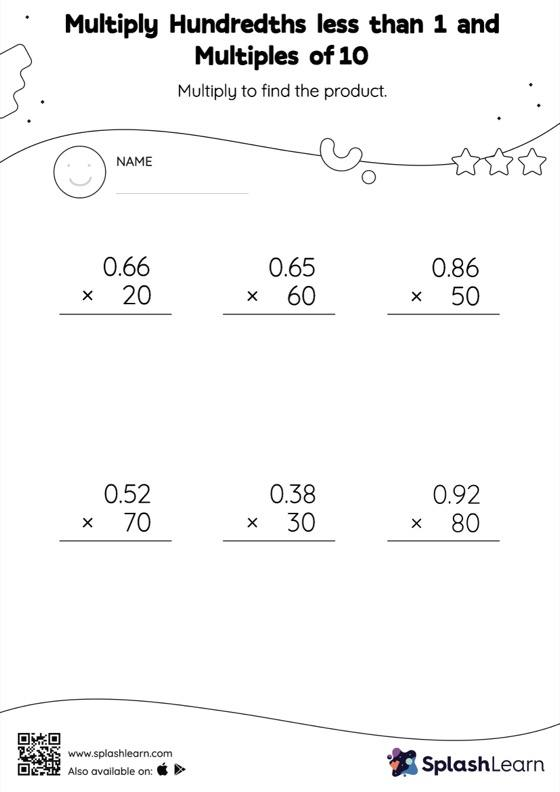# Multiply Hundredths less than 1 and Multiples of 10: Vertical Multiplication Worksheet

Home > Multiply Hundredths less than 1 and Multiples of 10: Vertical MultiplicationStudents understand that multiplying a decimal by a number that ends in zeros causes the decimal point to be placed in the product as many places from the right as the number of decimal digits in the multiplicand. In multiply hundredths less than 1 and multiples of 10 worksheet, they practice this idea in great detail. In this worksheet, students practice solving problems using the column method. This method is especially helpful with problems involving bigger multi-digit numbers as the format provides an easy structure to follow.